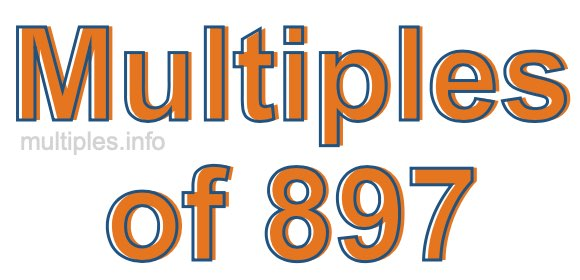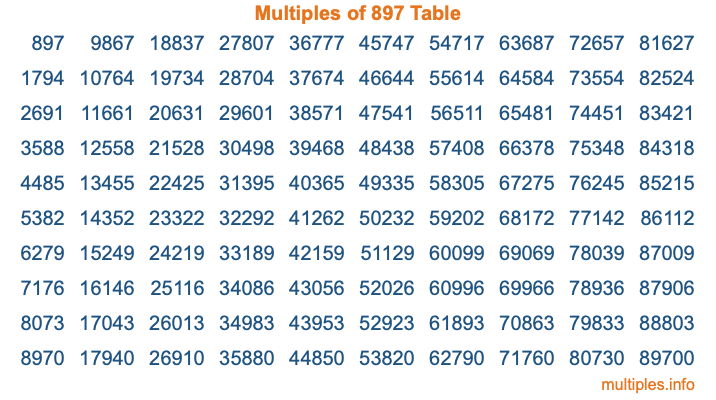Multiples of 897Welcome to the Multiples of 897 page. Here we will first teach you everything you will ever need to know about the multiples of 897, and then give you a study guide summary of everything we taught you to make sure you remember it all. Use this page to look up facts and learn information about the multiples of 897. This page will make you a multiples of eight hundred ninety-seven expert!

Definition of Multiples of 897
Multiples of 897 are all the numbers that when divided by 897 equal an integer. Each of the multiples of 897 are called a multiple. A multiple of 897 is created by multiplying 897 by an integer.

Therefore, to create a list of multiples of 897, you start with 1 multiplied by 897, then 2 multiplied by 897, then 3 multiplied by 897, and so on for as long as you want. Thus, the list of the first five multiples of 897 is 897, 1794, 2691, 3588, and 4485. To see a larger list of multiples of 897, see the printable image of Multiples of 897 further down on this page. We also have a category where you can choose any nth multiple of 897.

Multiples of 897 Checker
The Multiples of 897 Checker below checks to see if any number of your choice is a multiple of 897. In other words, it checks to see if there is any number (integer) that when multiplied by 897 will equal your number. To do that, we divide your number by 897. If the the quotient is an integer, then your number is a multiple of 897.

Is  a multiple of 897?

Least Common Multiple of 897 and ...
A Least Common Multiple (LCM) is the lowest multiple that two or more numbers have in common. This is also called the smallest common multiple or lowest common multiple and is useful to know when you are adding our subtracting fractions. Enter one or more numbers below (897 is already entered) to find the LCM.

Check out our LCM Calculator if you need more details about the Least Common Multiple or if you need the LCM for different numbers for adding and subtraction fractions.

nth Multiple of 897
As we stated above, 897 is the first multiple of 897, 1794 is the second multiple of 897, 2691 is the third multiple of 897, and so on. Enter a number below to find the nth multiple of 897.

th multiple of 897

Multiples of 897 vs Factors of 897
897 is a multiple of 897 and a factor of 897, but that is where the similarities end. All postive multiples of 897 are 897 or greater than 897. All positive factors of 897 are 897 or less than 897.

Below is the beginning list of multiples of 897 and the factors of 897 so you can compare:

Multiples of 897: 897, 1794, 2691, 3588, 4485, etc.

Factors of 897: 1, 3, 13, 23, 39, 69, 299, 897

As you can see, the multiples of 897 are all the numbers that you can divide by 897 to get a whole number. The factors of 897, on the other hand, are all the whole numbers that you can multiply by another whole number to get 897.

It's also interesting to note that if a number (x) is a factor of 897, then 897 will also be a multiple of that number (x).

Multiples of 897 vs Divisors of 897
The divisors of 897 are all the integers that 897 can be divided by evenly. Below is a list of the divisors of 897.

Divisors of 897: 1, 3, 13, 23, 39, 69, 299, 897

The interesting thing to note here is that if you take any multiple of 897 and divide it by a divisor of 897, you will see that the quotient is an integer.

Multiples of 897 Table
Below is an image of the first 100 multiples of 897 in a table. The table is in chronological order, column by column. The first column has the first ten multiples of 897, the second column has the next ten multiples of 897, and so on.The Multiples of 897 Table is also referred to as the 897 Times Table or Times Table of 897. You are welcome to print out our table for your studies.

Negative Multiples of 897
Although not often discussed or needed in math, it is worth mentioning that you can make a list of negative multiples of 897 by multiplying 897 by -1, then by -2, then by -3, and so on, to get the following list of negative multiples of 897:

-897, -1794, -2691, -3588, -4485, etc.

Multiples of 897 Summary
Below is a summary of important Multiples of 897 facts that we have discussed on this page. To retain the knowledge on this page, we recommend that you read through the summary and explain to yourself or a study partner why they hold true.

There are an infinite number of multiples of 897.

A multiple of 897 divided by 897 will equal a whole number.

897 divided by a factor of 897 equals a divisor of 897.

The nth multiple of 897 is n times 897.

The largest factor of 897 is equal to the first positive multiple of 897.

897 is a multiple of every factor of 897.

897 is a multiple of 897.

A multiple of 897 divided by a divisor of 897 equals an integer.

897 divided by a divisor of 897 equals a factor of 897.

Any integer times 897 will equal a multiple of 897.

Multiples of a Number
Here you can get the multiples of another number, all with the same attention to detail as we did for multiples of 897 on this page.

Multiples of
Multiples of 898
Did you find our page about multiples of eight hundred ninety-seven educational? Do you want more knowledge? Check out the multiples of the next number on our list!